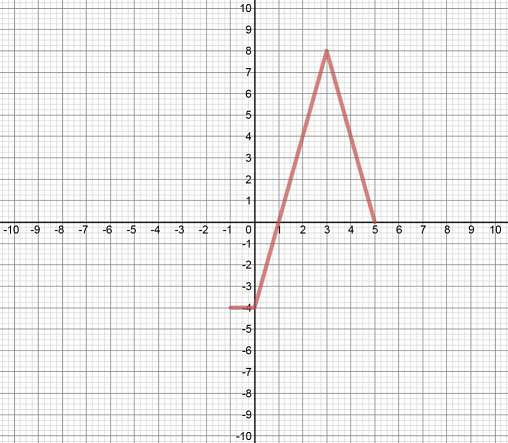# Combining transformations of functions#### Everything You Need in One Place

Homework problems? Exam preparation? Trying to grasp a concept or just brushing up the basics? Our extensive help & practice library have got you covered.#### Learn and Practice With Ease

Our proven video lessons ease you through problems quickly, and you get tonnes of friendly practice on questions that trip students up on tests and finals.#### Instant and Unlimited Help

Our personalized learning platform enables you to instantly find the exact walkthrough to your specific type of question. Activate unlimited help now!

0/5
##### Examples
###### Lessons
1. Describe the Combination of Transformations
Compared to $y = f\left( x \right)$, describe every step of transformations applied to:
$y = - 2f\left[ {3\left( {x + 4} \right)} \right] + 5$
1. Write the Equation of a Transformed Function
Transform the function $f\left( x \right) = \frac{1}{x}$ into the function $g\left( x \right)$ by:
1. stretching horizontally by a factor of 2 about the y-axis
2. stretching vertically by a factor of $\frac{3}{5}$ about the x-axis
3. vertical translation of 7 units up
4. reflection in the y-axis
5. horizontal translation of 4 units to the left
6. reflection in the x-axis

Write the function for $g(x)$.
2. Use "Coordinate Mapping Formula" to Graph a Transformed Function
Given the graph of $y = f\left( x \right)$ as shown,
1. describe every step of transformations applied to: $y = \frac{1}{4}f\left( {3 - \frac{x}{2}} \right) - 1$
2. Graph the transformed function on the same set of coordinate axes.
3. Shortcut: use "Coordinate Mapping Formula" to graph the transformed function.